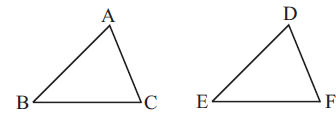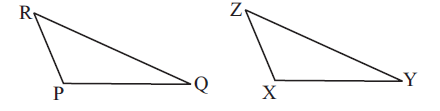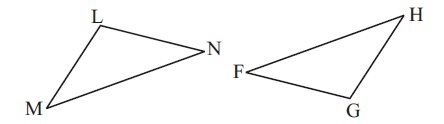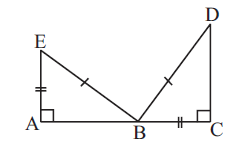# Ex.7.2 Q1 Congruence of Triangles - NCERT Maths Class 7

Go back to  'Ex.7.2'

## Question

Which congruence criterion do you use in the following?

 (a) Given: \begin{align}AC &= DF\\AB &= DE\\BC &= EF\end{align}                           So, $$ΔABC ≅ ΔDEF$$(b) Given:  \begin{align} ZX &= RP\\RQ &= ZY\\∠PRQ &= ∠XZY \end{align} So, $$ΔPQR ≅ ΔXYZ$$(c) Given: \begin{align} ∠MLN &= ∠FGH\\∠NML &= ∠GFH\\ML &= FG \end{align} So, $$ΔLMN ≅ ΔGFH$$(d) Given: \begin{align} EB &= DB\\AE &= BC\\∠A &= ∠C = 90^\circ \end{align} So, $$ΔABE ≅ ΔCDB$$Video Solution
Congruence Of Triangles
Ex 7.2 | Question 1

## Text Solution

(a)

What is known?In $$ΔABC$$ and $$ΔDEF$$

\begin{align} AC&= DF\\AB &= DE\\BC &= EF \end{align}

What is the unknown?

Congruence criterion by which these two triangles are congruent.

Reasoning:

Three sides of a $$ΔABC$$ are equal to the corresponding three sides of the other $$ΔDEF$$. So, $$SSS$$ congruence criterion can be used.

Steps:

Given, $$AC$$ = $$DF$$, $$AB = DE$$ and $$BC = EF$$. The three sides of a $$ΔABC$$ are equal to the corresponding three sides of the other $$ΔDEF$$. So, by $$SSS$$ congruence criterion, the two triangles are congruent.

(b)

What is known?\begin{align} ZX &= RP\\RQ &= ZY\\∠PRQ &= ∠XZY \end{align}

What is unknown?

Congruence criterion by which the two triangles are congruent.

Reasoning:

The two sides and one angle of a $$ΔPRQ$$ are equal to the corresponding two sides and one angle of the other $$ΔXYZ$$. So, $$SSS$$ congruence criterion can be used.

Steps:

Given,$$ZX = RP$$, $$RQ = ZY$$, $$∠PRQ = ∠XZY$$. The two sides and one angle of a triangle $$ΔPRQ$$ are equal to the corresponding two sides and one angle of the other triangle $$ΔXYZ$$. So, by $$SAS$$ congruence criterion, the two triangles are congruent.

(c)

What is known?\begin{align} ∠MLN&= ∠FGH\\∠NML &= ∠GFH\\ML &= FG \end{align}

What is the unknown?

By which congruence criterion two triangles are congruent.

Reasoning:

The two angles and one side of a triangle $$ΔLMN$$ are equal to the corresponding two sides and one angle of the other triangle $$ΔGFH$$. So, by using congruency criterion based on two angels and one side $$(ASA)$$ of triangles can be used.

Steps:

Given, $$∠MLN = ∠FGH$$, $$∠NML = ∠GFH$$ and $$ML = FG$$. The two sides and one angle of triangle $$ΔLMN$$ are equal to the two sides and one angle of the other triangle $$ΔGFH$$. So, by $$ASA$$ congruence criterion, the two triangles are congruent.

(d)

What is known?In $$ΔABE$$ and $$ΔCDB$$

\begin{align} EB &= DB\\AE &= BC\\∠A& = ∠C = 90^\circ \end{align}

What is the unknown?

By which congruence criterion two triangles are congruent.

Reasoning:

In this case, the hypotenuse and one side of a right-angled triangle are respectively equal to the hypotenuse and one side of another right-angled triangle. congruency is based on of a right-angle, hypotenuse and one side $$(RHS)$$ criterion.

Steps:

Since, $$EB = DB$$, $$AE = BC$$, $$∠A = ∠C = 90^\circ$$. The hypotenuse and one side of a right-angled triangle $$ΔABE$$ are equal to the hypotenuse and one side of the other right-angled triangle $$ΔCDB.$$ So, by $$(RHS)$$ congruence criterion, the two triangles are congruent.

Learn from the best math teachers and top your exams

• Live one on one classroom and doubt clearing
• Practice worksheets in and after class for conceptual clarity
• Personalized curriculum to keep up with school